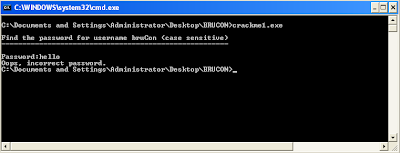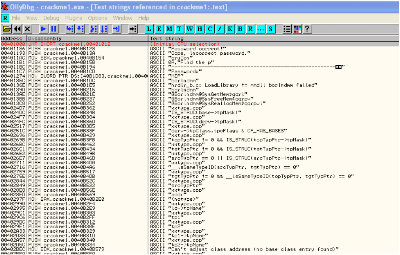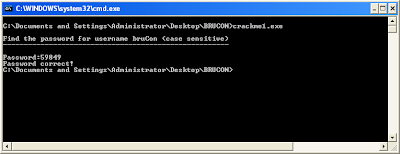## Monday, June 21, 2010

### Solutions: The Hex Factor v2009 (Level C200)

This is a straight-forward reverse engineering challenge. When analyzing the executable, we notice no packing was used by the coders. A simple tool like OllyDbg can tell this. This means we can jump right into the code without having to deal with exotic unpacking – good news!First things first: we launch the executable and immediately notice a prompt, asking for a password. The username is fixed at “bruCon” – this is already a huge help, since we can use this string to look for clues in the ASM code later on.

Enter a random password: we get the following error message:We get another useful clue when looking at the failed login screen: the message “Oops, incorrect password.” This is the second clue we get, and a second string we write down for later analysis in OllyDbg.

Next,we have a look at the literal text strings used in the executable from within OllyDbg:The two strings we remembered from running the exe are already mentioned as the first entries in the string table! (username “bruCon” and error message “Oops, incorrect password”).

Let’s trace jumps to the error message. We arrive at the following location in the assembly trace:Our string is mentioned at address 0x00401186. Why are we so interested in this error message? Well, whenever this error message is pushed on the stack from within the code, we can assume that somewhere close the keying algorithm is implemented (this is not always the case, but in this challenge, it is). So let’s have a look at what is happening in the assembly code just above this interesting error string.

Just above the call at 0x0040118B, we notice a short loop: since this is a fairly short executable, this loop could possibly be used by the keying algorithm to calculate the password – let’s have a closer look.

We set a breakpoint at 0x00401162, the entry point for the loop. Stepping through the code, we notice that each iteration is processing a different character of our username “bruCon” – a simple counter is incremented each iteration with the ASCII values for each individual character of the username, and this results in a total of 617 (decimal) – when stepping through the code, you will notice the register contains value 269, but this is of course the hexadecimal equivalent of 617.

After processing all characters and summing the ASCII values, we exit the loop at address 0x00401174. Next, the value of EDI (which contains 617 now) is multiplied by 61 – again, remember that OllyDbg is showing us hexadecimal numbers here!

The multiplication seems to be the last arithmetic operation used to calculate the password, so let’s give it a try. Our final result is 0x269 * 0x61 = 0xE9C9. Convert this to decimal, and we get 59849.

Launch the executable, let’s give this a try: bingo! We get a “Password correct!” message.Do you dare to take on The Hex Factor in 2010? Get your tickets now for BruCON (September, Brussels) or at SANS London (December, London)